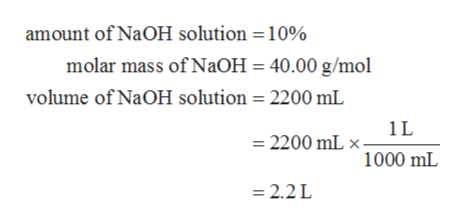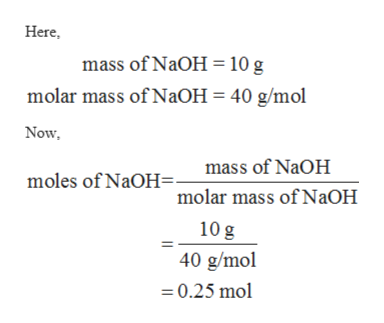# What is the molarity (M) of a(n) 10% NaOH solution (m.w. = 40.00 g/mol)?How many grams of NaOH would you need to make 2,200 mL of a 3 M NaOH solution (m.w. = 40.00 g/mol)?Can you explain in details, like what is the equation?

Question
86 views

What is the molarity (M) of a(n) 10% NaOH solution (m.w. = 40.00 g/mol)?

How many grams of NaOH would you need to make 2,200 mL of a 3 M NaOH solution (m.w. = 40.00 g/mol)?

Can you explain in details, like what is the equation?

check_circle

Step 1

The values are given as follows:help_outlineImage Transcriptioncloseamount of NaOH solution 10% molar mass of NaOH = 40.00 g/mol volume of NaOH solution 2200 mL 1L 2200 mL 1000 mL 2.2 L fullscreen
Step 2

Since, the solution of NaOH is 10 % which means 100 mL of solution has 10 g NaOH. Therefore, the number of moles of NaOH can be calculated as follows:help_outlineImage TranscriptioncloseHere mass of NaOH= 10 g molar mass of NaOH = 40 g/mol Now mass of NaOH moles of NaOH. molar mass of NaOH 10 g 40 g/mol 0.25 mol fullscreen
Step 3

The molarity of the NaOH solution c...

### Want to see the full answer?

See Solution

#### Want to see this answer and more?

Solutions are written by subject experts who are available 24/7. Questions are typically answered within 1 hour.*

See Solution
*Response times may vary by subject and question.
Tagged in

### General Chemistry